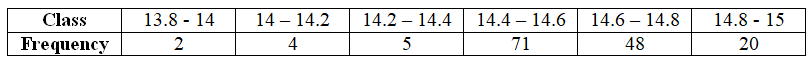The times, in seconds, taken by 150 athletes to run a 110m hurdle race are tabulated below:From the following choices, the number of athletes who completed the race in less than 14.6 seconds is:

# The times, in seconds, taken by athletes to run a hurdle race are tabulated below:From the following choices, the number of athletes who completed the race in less than seconds is:

1. A
2. B
3. C
4. D

Fill Out the Form for Expert Academic Guidance!l

+91

Live ClassesBooksTest SeriesSelf Learning

Verify OTP Code (required)

### Solution:

It is given that, a table of data about times in seconds, taken by athletes to run a hurdle race.
The number of athletes who completed the race in less than seconds can be obtained by adding the frequencies .
By adding the frequency, we get,

The number of athletes completes the race is seconds is .
Hence, option 3) is correct.

## Related content

 Area of Square Area of Isosceles Triangle Pythagoras Theorem Triangle Formula Perimeter of Triangle Formula Area Formulae Volume of Cone Formula Matrices and Determinants_mathematics Critical Points Solved Examples Type of relations_mathematics+91

Live ClassesBooksTest SeriesSelf Learning

Verify OTP Code (required)